## JAC Class 9 Maths Solutions Chapter 2 Polynomials Ex 2.2

Jharkhand Board JAC Class 9 Maths Solutions Chapter 2 Polynomials Ex 2.2 Textbook Exercise Questions and Answers.

## JAC Board Class 9th Maths Solutions Chapter 2 Polynomials Ex 2.2

Page – 34

Question 1.
Find the value of the polynomial 5x + 4x2 + 3 at
(i) x = 0
(ii) x = – 1
(iii) x = 2 ,
(i) p(x) = 5x + 4x2 + 3
p(0) = 5(0) + 4(0)2 + 3 = 3

(ii) p(x) = 5x + 4x2 + 3
P(-1) = 5(-1) + 4(-1)2 + 3
= -5 + 4(1) + 3 = 2

(iii) p(x) = 5x + 4x2 + 3
p(2) = 5(2) + 4(2)2 + 3
= 10 + 16 + 3 = 29

Question 2.
Find p(0), p(1) and p(2) for each of the following polynomials:
(i) p(y) = y2 – y + 1
(ii) p(x) = x3
(iii) p(t) = 2 + t + 2t2 – t3
(iv) p(x) = (x – 1) (x + 1)
(i) p(y) = y2 – y + 1
P(0) = (0)2 – (0) + 1 = 1
p(1) = (1)2 – (1) + 1 = 1
p(2) = (2)2 – (2) + 1 = 3

(ii) p(x) = x3
P(0) = (0)3 = 0
p(1) = (1)3 = 1
p(2) = (2)3 = 8

(iii) p(t) = 2 + t + 2t2 – t3
p(0) = 2 + 0 + 2 (0)2 – (0)3 = 2
P(1) = 2 + (1) + 2(1)2 – (1)3
= 2 + 1 + 2 – 1 = 4
p(2) = 2 + 2 + 2(2)2 – (2)3
= 2 + 2 + 8 – 8 = 4

(iv) p(x) = (x – 1) (x + 1)
P(0) = (0 – 1) (0 + 1) = (-1) (1) = – 1
p(1) = (1 – 1) (1 + 1) = 0(2) = 0
p(2) = (2 – 1) (2 + 1) = 1(3) = 3Page – 35

Question 3.
Verify whether the following are zeroes of the polynomial, indicated against them.
(i) p(x) = 3x + 1, x = $$– \frac{1}{3}$$
(ii) p(x) = 5x – π, x = $$\frac{4}{5}$$
(iii) p(x) = x2 – 1, x = 1, – 1
(iv) p(x) = (x + 1) (x – 2), x = – 1, 2
(v) p(x) = x2, x = 0
(vi) p(x) = lx + m, x = $$– \frac{m}{l}$$
(vii) p(x) = 3x2 – 1, x = $$-\frac{1}{\sqrt{3}}, \frac{2}{\sqrt{3}}$$
(viii) p(x) = 2x + 1, x = $$\frac{1}{2}$$
(i) If x = $$– \frac{1}{3}$$ is a zero of polynomial p(x) then p($$– \frac{1}{3}$$) = 0
p($$– \frac{1}{3}$$) = 3($$– \frac{1}{3}$$) + 1 = -1 + 1 = 0
Therefore, x = $$– \frac{1}{3}$$ is a zero of polynomial p(x) = 3x + 1.

(ii) If x = $$\frac{4}{5}$$ is a zero of polynomial
p(x) = 5x – π then p($$\frac{4}{5}$$) should be 0.
p($$\frac{4}{5}$$) = 5($$\frac{4}{5}$$) – π = 4 – π ≠ 0
Therefore, x = $$\frac{4}{5}$$ is not a zero of given polynomial p(x) = 5x – π.

(iii) If x = 1 and x = – 1 are zeroes of polynomial p(x) = x2 – 1, then p(1) and p(-1) each should be 0.
p(1) = (1)2 – 1 = 0 and
P(-1) = (-1)2 – 1 = 0
Hence, x = 1 and – 1 are zeroes of the polynomial p(x) = x2 – 1.

(iv) If x = -1 and x = 2 are zeroes of polynomial p(x) = (x + 1) (x – 2), then p( -1) and p(2) each should be 0.
p(-1) = (-1 + 1) (-1 – 2) = 0 (-3) = 0, and
p(2) = (2 + 1) (2 – 2) = 3 (0) = 0
Therefore, x = – 1 and x = 2 are zeroes of the polynomial p(x) = (x+1) (x – 2).(v) If x = 0 is a zero of polynomial p(x) = x2, then p(0) should be zero.
Here, p(0) = (0)2 = 0
Hence, x = 0 is a zero of the polynomial p(x) = x2.

(vi) If x = $$– \frac{m}{l}$$ is a zero of polynomial p(x) = lx + m, then p($$– \frac{m}{l}$$)= 0 should be 0.
p($$– \frac{m}{l}$$) = l($$– \frac{m}{l}$$) + m = -m + m = 0
Therefore x = ($$– \frac{m}{l}$$) is a zero of polynomial p(x) = lx + m

(vii) If x = $$-\frac{1}{\sqrt{3}}$$ and x = $$\frac{2}{\sqrt{3}}$$ are zeros of polynomial p(x) = 3x2 – 1, then p($$-\frac{1}{\sqrt{3}}$$) and p($$\frac{2}{\sqrt{3}}$$)should be 0.
p($$\frac{-1}{\sqrt{3}}$$) = $$3\left(\frac{-1}{\sqrt{3}}\right)^2$$ – 1 = 3($$\frac{1}{3}$$)-1 = 1 – 1 = 0
p($$\frac{2}{\sqrt{3}}$$) = $$3\left(\frac{2}{\sqrt{3}}\right)^2$$ – 1 = 3($$\frac{4}{3}$$) – 1 = 4 – 1 = 3 ≠ 0
Therefore, x = $$\frac{-1}{\sqrt{3}}$$ is a zero of polynomial p(x) = 3x2 – 1 but, x = $$\frac{2}{\sqrt{3}}$$ is not a zero of this polynomial.

(viii) If x = $$\frac{1}{2}$$ is a zero of polynomial p(x) = 2x + 1then p($$\frac{1}{2}$$) should be 0.
p($$\frac{1}{2}$$) = 2($$\frac{1}{2}$$) + 1 = 1 + 1 = 2 ≠ 0
Therefore, x = $$\frac{1}{2}$$ is not a zero of given polynomial p(x) = 2x + 1

Question 4.
Find the zero of the polynomial in each of the following cases:
(i) p(x) = x + 5
(ii) p(x) = x – 5
(iii) p(x) = 2x + 5
(iv) p(x) = 3x – 2
(v) p(x) = 3x
(vi) p(x) = ax, a ≠ 0
(vii) p(x) = cx + d, c ≠ 0, d, are real numbers.
(i) p(x) = x + 5
p(x) = 0
x + 5 = 0
x = – 5
Therefore, x = – 5 is a zero of polynomial p(x) = x + 5 .

(ii) p(x) = x – 5
p(x) =0
x – 5 = 0
x = 5
Therefore, x = 5 is a zero of polynomial p(x) = x – 5.(iii) p(x) = 2x + 5
p(x) = 0
2x + 5 = 0
2x = – 5
x = $$– \frac{5}{2}$$
Therefore, x = $$– \frac{5}{2}$$ is a zero of polynomial p(x) = 2x + 5.

(iv) p(x) = 3x – 2
p(x) = 0
3x – 2 = 0
x = $$\frac{2}{3}$$
Therefore, x = $$\frac{2}{3}$$ is a zero of polynomial p(x) = 3x – 2.

(v) p(x) = 3x
p(x) = 0
3x = 0
x = 0
Therefore, x = 0 is a zero of polynomial p(x) = 3x.

(vi) p(x) = ax, a ≠ 0
p(x) = 0
ax = 0
x = 0
Therefore, x = 0 is a zero of polynomial p(x) = ax.

(vii) p(x) = cx + d, c ≠ 0
p(x) = 0
cx + d = 0
x = $$– \frac{d}{c}$$
Therefore, x = $$– \frac{d}{c}$$ is a zero of polynomial p(x) = cx + d.

## JAC Class 9 Maths Solutions Chapter 2 Polynomials Ex 2.1

Jharkhand Board JAC Class 9 Maths Solutions Chapter 2 Polynomials Ex 2.1 Textbook Exercise Questions and Answers.

## JAC Board Class 9th Maths Solutions Chapter 2 Polynomials Ex 2.1

Question 1.
Which of the following expressions are polynomials in one variable and which are not? State reasons for your answer.
(i) 4x2 – 3x + 7
(ii) y2 + $$\sqrt{2}$$
(iii) 3$$\sqrt{t}$$ + t$$\sqrt{2}$$
(iv) y + $$\frac{2}{y}$$
(v) x10 + y3 + t50
(i) 4x2 – 3x + 7
There is only one variable x with whole number powers so this is a polynomial in one variable.

(ii) y2 + $$\sqrt{2}$$
There is only one variable y with whole number powers so this is a polynomial in one variable.

(iii) 3$$\sqrt{t}$$ + t$$\sqrt{2}$$
There is only one variable t but in 3$$\sqrt{t}$$ power of t is $$\frac{1}{2}$$ which is not a whole number. So, 3$$\sqrt{t}$$ + t$$\sqrt{2}$$ is not a polynomial.

(iv) y + $$\frac{2}{y}$$
There is only one variable y but $$\frac{2}{y}$$ = 2y-1 So, the power of y is not a whole number in the second term. Hence, y + $$\frac{2}{y}$$ is not a polynomial.

(v) x10 + y3 + t50
There are three variables x, y and t. So, this is not a polynomial in one variable.

Question 2.
Write the coefficients of x2 in each of the following:
(i) 2 + x2 + x
(ii) 2 – x2 + x3
(iii) $$\frac{π}{2}$$x2 + x
(iv) $$\sqrt{2}$$ – 1
(i) Coefficient of x2 = 1
(ii) Coefficient of x2 = -1
(iii) Coefficient of x2 = $$\frac{π}{2}$$
(iv) Coefficient of x2 = 0Question 3.
Give one example each of a binomial of degree 35, and of a monomial of degree 100.
3x35 + 7 and 4x100 are binomials of degree 35 and monomial of degree 100 respectively.

Question 4.
Write the degree of each of the following polynomials:
(i) 5x3 + 4x2 + 7x
(ii) 4 – y2
(iii) 5t – $$\sqrt{7}$$
(iv) 3
(i) 5x3 has highest power in the given polynomial, which is 3. Therefore, degree of polynomial is 3.
(ii) -y2 has highest power in the given polynomial, which is 2. Therefore, degree of polynomial is 2.
(iii) 5t has highest power in the given polynomial, which is 1. Therefore, degree of polynomial is 1.
(iv) There is no variable in the given polynomial. Therefore, degree of polynomial is 0.

Question 5.
Classify the following as linear, quadratic and cubic polynomials:
(i) x2 + x
(ii) x – x3
(iii) y + y2 + 4
(iv) 1 + x
(v) 3t
(vi) r2
(vii) 7x3
(i) x2 + x Quadratic Polynomial
(ii) x – x3 Cubic Polynomial
(iii) y + y2 + 4 Quadratic Polynomial
(iv) 1 + x Linear Polynomial
(v) 3t Linear Polynomial
(vii) 7x3 Cubic Polynomial

## JAC Class 9 Maths Solutions Chapter 1 Number Systems Ex 1.6

Jharkhand Board JAC Class 9 Maths Solutions Chapter 1 Number Systems Ex 1.6 Textbook Exercise Questions and Answers.

## JAC Board Class 9th Maths Solutions Chapter 1 Number Systems Exercise 1.6

Question 1.
Find:
(i) $$64^{\frac{1}{2}}$$
(ii) $$32^{\frac{1}{5}}$$
(iii) $$125^{\frac{1}{3}}$$
(i) $$64^{\frac{1}{2}}=\left(2^6\right)^{\frac{1}{2}}=2^{6 \times \frac{1}{2}}=2^3=8$$
(ii) $$32^{\frac{1}{5}}=\left(2^5\right)^{\frac{1}{5}}=2^{5 \times \frac{1}{5}}=2$$
(iii) $$125^{\frac{1}{3}}=\left(5^3\right)^{\frac{1}{3}}=5^{3 \times \frac{1}{3}}=5$$

Question 2.
Find:
(i) $$9^{\frac{3}{2}}$$
(ii) $$32^{\frac{2}{5}}$$
(iii) $$16^{\frac{3}{4}}$$
(iv) $$125^{\frac{-1}{3}}$$
(i) $$9^{\frac{3}{2}}=\left(3^2\right)^{\frac{3}{2}}=3^3=27$$
(ii) $$32^{\frac{2}{5}}=\left(2^5\right)^{\frac{2}{5}}=2^2=4$$
(iii) $$16^{\frac{3}{4}}=\left(2^4\right)^{\frac{3}{4}}=2^3=8$$
(iv) $$125^{\frac{-1}{3}}=\frac{1}{(125)^{\frac{1}{3}}}=\frac{1}{\left(5^3\right)^{\frac{1}{3}}}=\frac{1}{5}$$Question 3.
(i) $$2^{\frac{2}{3}} \cdot 2^{\frac{1}{5}}$$
(ii) $$\left(\frac{1}{3^3}\right)^7$$
(iii) $$\frac{11^{\frac{1}{2}}}{11^{\frac{1}{4}}}$$
(iv) $$7^{\frac{1}{2}} \cdot 8^{\frac{1}{2}}$$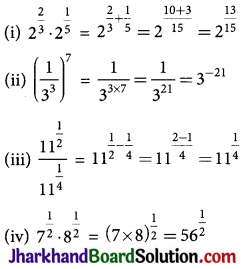## JAC Class 9 Maths Solutions Chapter 1 Number Systems Ex 1.5

Jharkhand Board JAC Class 9 Maths Solutions Chapter 1 Number Systems Ex 1.5 Textbook Exercise Questions and Answers.

## JAC Board Class 9th Maths Solutions Chapter 1 Number Systems Exercise 1.5

Question 1.
Classify the following numbers as rational or irrational:
(i) 2 – $$\sqrt{5}$$
(ii) (3 + $$\sqrt{23}$$) – $$\sqrt{23}$$
(iii) $$\frac{2 \sqrt{7}}{7 \sqrt{7}}$$
(iv) $$\frac{1}{\sqrt{2}}$$
(v) 2π
(i) 2 – $$\sqrt{5}$$ = 2 – 2.2360679…
= -0.2360679…
Since the number is non-terminating non-recurring therefore, it is an irrational number.

(ii) (3 + $$\sqrt{23}$$) – $$\sqrt{23}$$
3 + $$\sqrt{23}$$ – $$\sqrt{23}$$
= 3 = $$\frac{3}{1}$$
The number is rational number as it can represented in $$\frac{p}{q}$$ form, where p, q ∈ Z, q ≠ 0

(iii) $$\frac{2 \sqrt{7}}{7 \sqrt{7}}$$
The number is rational number as it can represented in $$\frac{p}{q}$$ form, where p, q ∈ Z, q ≠ 0

(iv) $$\frac{1}{\sqrt{2}}$$
= 0.7071067811…
Since the number is non-terminating non-recurring therefore, it is an irrational number.

(v) 2π = 2 × 3.1415… = 6.2830…
Since the number is non-terminating non-recurring therefore, it is an irrational number.

Question 2.
Simplify each of the following expressions:
(i) (3 + $$\sqrt{3}$$) (2 + $$\sqrt{2}$$)
(ii) (3 + $$\sqrt{3}$$) (3 – $$\sqrt{3}$$)
(iii) ($$\sqrt{5}$$ + $$\sqrt{2}$$)2
(iv) ($$\sqrt{5}$$ – $$\sqrt{2}$$)($$\sqrt{5}$$ + $$\sqrt{2}$$)
(i) (3 + $$\sqrt{3}$$) (2 + $$\sqrt{2}$$)
= 3 × 2 + 2$$\sqrt{3}$$ + 3$$\sqrt{2}$$ + $$\sqrt{3}$$ ×$$\sqrt{2}$$
= 6 + 2$$\sqrt{3}$$ + 3$$\sqrt{2}$$ + $$\sqrt{6}$$

(ii) (3 + $$\sqrt{3}$$) (3 – $$\sqrt{3}$$)
= 32– ($$\sqrt{3}$$)2 = 9 – 3 = 6
[∵ (a + b) (a – b) = a2 – b2]

(iii) ($$\sqrt{5}$$ + $$\sqrt{2}$$)2
= ($$\sqrt{5}$$)2 + ($$\sqrt{2}$$)2 + 2 × $$\sqrt{5}$$ × $$\sqrt{2}$$
[∵ (a + b)2 = a2 + b2 + 2ab]
= 5 + 2 + 2 × $$\sqrt{5}$$ ×$$\sqrt{2}$$
= 7 + 2$$\sqrt{10}$$

(iv) ($$\sqrt{5}$$ – $$\sqrt{2}$$)($$\sqrt{5}$$ + $$\sqrt{2}$$)
= ($$\sqrt{5}$$)2 – ($$\sqrt{2}$$)2
= 5 – 2 = 3
[∵ (a + b) (a – b) = a2 – b2]Question 3.
Recall, 7t is defined as the ratio of the circumference (say c) of a circle to its diameter (say d). That is, n = c/d. This seems to contradict the fact that n is irrational. How will you resolve this contradiction?
There is no contradiction. When we measure a value with a scale, we only obtain an approximate value. We never obtain an exact value. Therefore, we may not realise that either c or d is irrational. The value of n is almost equal to 22/7 or 3.14159…

Question 4.
Represent $$\sqrt{9.3}$$ on the number line.
Step 1: Draw a line segment AB of 9.3 units. Extend it to C so that BC is of 1 unit.
Step 2: Now, AC = 10.3 units. Find the midpoint of AC and name it as O.
Step 3: Draw a semicircle with radius OC and centre O.
Step 4: Draw a perpendicular line segment BD to AC at point B which intersects the semicircle at D. Also, Join OD.
Step 5: Now, OBD is a right angled triangle.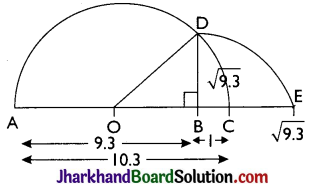Here, OD = 10.3/2 (radius of semicircle),
OC = $$\frac{10.3}{2}$$, BC = 1
OB = OC – BC = ($$\frac{10.3}{2}$$) – 1 = $$\frac{8.3}{2}$$
Using Pythagoras theorem
OD2 = BD2 + OB2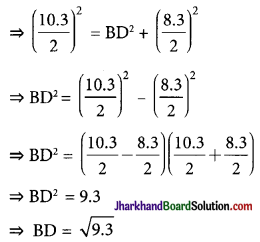Thus, the length of BD is $$\sqrt{9.3}$$ units.

Step 6: Taking BD as radius and B as centre draw an arc which touches the line segment AC extended at the point E. The point E is at a distance of $$\sqrt{9.3}$$ from B as shown in the figure.Question 5.
Rationalise the denominators of the following:
(i) $$\frac{1}{\sqrt{7}}$$
(ii) $$\frac{1}{\sqrt{7}-\sqrt{6}}$$
(iii) $$\frac{1}{\sqrt{5}+\sqrt{2}}$$
(iv) $$\frac{1}{\sqrt{7}-2}$$
(i) $$\frac{1}{\sqrt{7}}$$ = $$\frac{1 \times \sqrt{7}}{\sqrt{7} \times \sqrt{7}}$$ = $$\frac{\sqrt{7}}{7}$$

(ii) $$\frac{1}{\sqrt{7}-\sqrt{6}}$$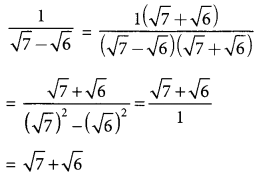(iii) $$\frac{1}{\sqrt{5}+\sqrt{2}}$$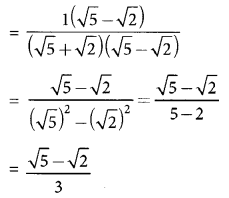(iv) $$\frac{1}{\sqrt{7}-2}$$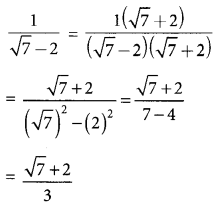## JAC Class 10 Sanskrit व्याकरणम् संख्याज्ञानम्

Jharkhand Board JAC Class 10 Sanskrit Solutions व्याकरणम् संख्याज्ञानम् Questions and Answers, Notes Pdf.

## JAC Board Class 10th Sanskrit व्याकरणम् संख्याज्ञानम्

संख्या-ज्ञान – इस संसार में सभी लोगों के लिए संख्या (अंकों) का ज्ञान परम आवश्यक है क्योंकि संख्या के ज्ञान के बिना हमारे जीवन में आदान-प्रदान का कोई भी कार्य सम्भव नहीं हो सकता है। अतः हम यहाँ 100 से आगे की संख्याओं (अंकों) का ज्ञान प्राप्त करेंगे।

हिन्दी अर्थ सहित संख्यावाची शब्द :

यहाँ कुछ पारिभाषिक संस्कृत शब्दों का हिन्दी अर्थ विद्यार्थियों के हित को ध्यान में रखते हुए दिया जा रहा है –

(i) शतम् – सौ
(ii) सहस्रम् – हजार
(iii) अयुतम् – दस हजार
(iv) लक्षम् – लाख
(v) प्रयुतम्/नियुतम् – दस लाख
(vi) कोटि: – करोड़
(vii) दशकोटि: – दस करोड़
(viii) अर्बुदम् – अरब
(ix) दशार्बुदम् – दस अरब
(x) खर्वम् – खरब
(xi) दशखर्वम् – दस खरब
(xii) नीलम् – नील
(xiii) दशनीलम् – दस नील
(xiv) पद्मम् – पद्म
(xv) दशपद्मम् – दस पद्म
(xvi) शंखम् – शंख
(xvii) दशशंखम्-दस शंख
(xviii) महाशंखम्-महाशंख

शतम् (100) से ऊपर के संख्यावाचक शब्द बनानानियम – (i) ‘शत’ (100) आदि संख्यावाचक शब्दों के साथ लघु (छोटी) संख्या के मिलाने के लिए लघु (छोटी) संख्या के साथ ‘अधिक’ या ‘उत्तर’ शब्द वृहत्तर (बड़ी) संख्या के पहले लगा दिया जाता है। यथा-‘एक सौ तेरह’; यहाँ लघु संख्या तेरह है, इसकी संस्कृत है-‘त्रयोदश’। इसके आगे अधिक लगाकर इसके बाद वृहत्तर (बड़ी) संख्या ‘शतम्’ लगाने से ‘एक सौ तेरह’ की संस्कृत हुई- “त्रयोदशाधिकशतम्”।

(ii) शत् (100) सहस्र (1000) इत्यादि संख्याओं के साथ यदि उनका आधा (50, 500 आदि) हो तो सार्धं (आधा सहित), चौथाई साथ हो (25, 250 आदि) तो ‘सपादम्’ (चौथाई साथ) और चौथाई ‘कम हो तो’ पादोन (चौथाई कम) शब्द का उनके साथ प्रयोग किया जाता है। यथा-450 ‘सार्द्ध-शत-चतुष्टयम्” (आधे सहित सौ चार) इसी प्रकार 125 को ‘सपादशतम्’ (चौथाई सहित सौ) लिखते हैं। 1750 को ‘पादोनसहस्रद्वयम्’ (चौथाई कम दो हजार) लिखा जायेगा।

विशेष-शत, सहस्र इत्यादि के पहले द्वि, त्रि आदि के आने पर, समाहार द्विगु हो जाने से वे विशेषण नहीं रहते, क्योंकि समाहार द्विगु हो जाने पर वे विशेष्य पद हो जाते हैं। यथा-द्विशती (200), त्रिशती (300) आदि।

(iii) अवयव दिखाने के लिए द्वय, त्रय, चतुष्टय, पञ्चक, षट्क, सप्तक, अष्टक इत्यादि ‘क’ प्रत्ययान्त एकवचनान्त नपुंसकलिंग शब्दों का प्रयोग किया जाता है।

(iv) संख्यावाचक शब्दों के प्रयोग करने में यदि संशय हो तो संख्यावाचक शब्द के साथ ‘संख्यक’ शब्द लगाकर अकारान्त पद की तरह रूप चलाकर सरलता से अनुवाद किया जा सकता है।

ध्यातव्य – संस्कृत में संख्या को उल्टे क्रम से लिखना शुरू करते हैं जैसे-101 में, पहले एक को फिर सौ को लिखेंगे। बीच में ज्यादा का शब्द ‘अधिक’ लिखेंगे। याद करें हमारे पुराने बुजुर्ग लोग कैसे गिनती बताते थे। 130 को = तीस ज्यादा सौ, 19 को = एक कम बीस, 540 को = चालीस ज्यादा पाँच सौ, यही तरीका संस्कृत का है। जैसे –

1. 101 = उल्टाक्रम = एक अधिक सौ = एक + अधिकशतम् = एकाधिकशतम्।
2. 140 = उल्टाक्रम = चालीस अधिक सौ = चत्वारिंशत् + अधिकशतम् = चत्वारिंशदधिकशतम्।
3. 165 = उल्टाक्रम = पाँच साठ अधिक सौ = पंच षष्टि + अधिक शतम् = पंचषष्ट्यिधिकशतम्।
4. 300 = तीन सौ = त्रिशतम्।
5. 1965 = उल्टाक्रम = पाँच साठ अधिक एक कम बीस सौ = पंचषष्टि + अधिक + एकोनविंशतिशतम् = पंचषष्ट्य धिकैकोनविंशतिशतम्
6. 2000 = दो हजार = द्विसहस्रम्उपर्युक्त नियम के अनुसार 100 (शतम्) से ऊपर के संख्यावाचक शब्द –

101. एकाधिकशतम्
102. यधिकशतम्
103. त्र्यधिकशतम्
104. चतुरधिकशतम्
105. पञ्चाधिकशतम्
106. षडधिकशतम्
107. सप्ताधिकशतम्
108. अष्टाधिकशतम्
109. नवाधिकशतम्
110. दशाधिकशतम्
111. एकादशाधिकशतम्
112. द्वादशाधिकशतम्
113. त्रयोदशाधिकशतम्
114. चतुर्दशाधिकशतम्
115. पञ्चदशाधिकशतम्
116. षोडशाधिकशतम्
117. सप्तदशाधिकशतम्
118. अष्टादशाधिकशतम्
119. नवदशाधिकशतम्
120. विंशत्यधिकशतम्
121. एकविंशत्यधिकशतम्
122. द्वारिंशत्यधिकशतम्
123. ज्योविंशत्यधिकशतम्
124. चतुर्विंशत्यधिकशतम्
125. पञ्चविंशत्यधिकशतम्
126. षड्विंशत्यधिकशतम्
127. सप्तविंशत्यधिकशतम्
128. अष्टाविंशत्यधिक शतम्
129. नवविंशत्यधिकशतम्
130. त्रिंशदधिकशतम्
131. एकत्रिंशदधिकशतम्
132. द्वात्रिंशदधिकशतम्।
133. त्रयस्त्रिंशदधिकशतम्
134. चतुत्रिंशदधिकशतम्
135. पञ्चत्रिंशदधिकशतम्
136. षट्त्रिंशदधिकशतम्
137. सप्तत्रिंशदधिकशतम्
138. अष्टत्रिंशदधिकशतम्
139. नवत्रिंशदधिकशतम्
140. चत्वारिंशदधिकशतम्
141. एकचत्वारिंशदधिकशतम्
142. द्विचत्वारिंशदधिकशतम्
143. त्रिचत्वारिंशदधिकशतम्
144. चतुश्चत्वारिंशदधिकशतम्
145. पञ्चचत्वारिंशदधिकशतम्
146. षट्चत्वारिंशदधिकशतम्
147. सप्तचत्वारिंशदधिकशतम
148. अष्टचत्वारिंशदधिकशतम्
149. नवचत्वारिंशदधिकशंतम्
150. पञ्चाशदधिकशतम्
151. एकपञ्चाशदधिकशतम्
152. द्विपञ्चाशदधिकशतम्
153. त्रिपञ्चाशदधिकशतम्
154. चतुःपञ्चाशदधिकशतम्
155. पञ्चपञ्चाशदधिकशतम्
156. षट्पञ्चाशदधिकशतम्
157. सप्तपञ्चाशदधिकशतम्
158. अष्टपञ्चाशदधिकशतम्
159. नवपञ्चाशदधिकशतम्
160. षष्ट्यधिकशतम्
161. एकषष्ट्यधिकशतम्
162. द्विषष्ट्यधिकशतम्
163. त्रिषष्ट्यधिकशतम्
164. चतुःषष्ट्यधिकशतम्
165. पञ्चषष्ट्यधिकशतम्
166. षट्षष्ट्यधिकशतम्
167. सप्तषधिकशतम्
168. अष्टषष्ट्यधिकशतम्
169. नवषष्ट्यधिकशतम्
170. सप्तत्यधिकशतम्
171. एकसप्तत्यधिकशतम्
172. द्विसप्तत्यधिकशतम्
173. त्रिसप्तत्यधिकशतम्
174. चतुःसप्तत्यधिकशतम्
175. पञ्चसप्तत्यधिकशतम्
176. षष्टसप्तत्यधिकशतम्
177. सप्तसप्तत्यधिकशतम्
178. अष्टसप्तत्यधिकशतम्
179. नवसप्तत्यधिकशतम्
180. अशीत्यधिकशतम्।
181. एकाशीत्यधिकशतम्
182. द्वयशीत्यधिकशतम्
183. व्यशीत्यधिक शतम्
184. चतुरशीत्यधिकशतम्
185. पञ्चाशीत्यधिकशतम्
186. षडशीत्यधिकशतम्
187. सप्ताशीत्यधिकशतम्
188. अष्टाशीत्यधिकशतम्
189. नवाशीत्यधिकशतम्
190. नवत्यधिकशतम्
191. एकनवत्यधिकशतम्
192. द्विनवत्यधिकशत्
193. त्रिनवत्यधिकशतम्
194. चतुर्नवत्यधिकशतम्
195. पञ्चनवत्यधिकशतम्
196. षण्णवत्यधिकशतम्
197. सप्तनवत्यधिकशतम्
198. अष्टनवत्यधिकशतम्
199. नवनवत्यधिकशतम्
200. द्विशती द्विशतम्/द्विशतकम् शतद्वयम् इसी प्रकार
201 (एकाधिकद्विशती) आदि संख्याएँ लिखी जायेंगी। उपर्युक्त नियम के अनुसार
200 के ऊपर संख्यावाचक शब्द
201 एकाधिकद्विशतम्।
301 एकाधिकत्रिशतम्।
401 एकाधिकचतुःशतम्/एकोत्तरचतुःशतम्/एकाधिकं चतुःशतम्/एकोत्तरं चतुःशतम्।
500 पञ्चशतम्/शतपञ्चकम्/पञ्चशतकम्।
501 एकाधिकपञ्चशतम्/एकोत्तरपञ्चशतम्/एकाधिकं पञ्चशतम्/एकोत्तरं पञ्चशतम्।
502 यधिकपञ्चशतम्/द्वयुत्तरपञ्चशतम्/यधिकं पञ्चशतम्/द्युत्तरं पञ्चशतम्।
503 व्यधिकपञ्चशतम्/व्युत्तरपञ्चशतम्/त्र्यधिकं पञ्चशतम्/युत्तरं पञ्चशतम्।
504 चतुरधिकपञ्चशतम्/चतुरुत्तरपञ्चशतम्/चतुरधिकं पञ्चशतम्/चतुरुत्तरं पञ्चशतम्।
505 पञ्चाधिकपञ्चशतम्/पञ्चोत्तरपञ्चशतम्/पञ्चाधिकं पञ्चशतम्/पञ्चोत्तरं पञ्चशतम्।
506 षडधिकपञ्चशतम्/षडुत्तरपञ्चशतम्/षडधिकं पञ्चशतम्/षडुत्तरं पञ्चशतम्।
507 सप्ताधिकपञ्चशतम्/सप्तोत्तरपञ्चशतम्/सप्ताधिकं पञ्चशतम्/सप्तोत्तरं पञ्चशतम्।
508 अष्टाधिकपञ्चशतम्/अष्टोत्तरपञ्चशतम्/अष्टाधिकं पञ्चशतम्/अष्टोत्तरं पञ्चशतम्।
509 नवाधिकपञ्चशतम्/नवोत्तरपञ्चशतम्/नवाधिकं पञ्चशतम्/नवोत्तरं पञ्चशतम्।
510 दशाधिकपञ्चशतम्/दशोत्तरपञ्चशतम्/दशाधिकं पञ्चशतम्/दशोत्तरं पञ्चशतम्।
517 सप्तदशाधिकपञ्चशतम्/सप्तदशोत्तरपञ्चशतम्।सप्तदशाधिकं पञ्चशतम्/सप्तदशोत्तरं पञ्चशतम्।
600 षट्शतम्/शतषट्कम्/षट्शतकम्।
625 पञ्चविंशत्यधिकषट्शतम्/पञ्चविंशत्यधिकं षट्शतम्/पञ्चविंशत्युत्तरषट्शतम्/पञ्चविंशत्युत्तरं षट्शतम्।
637 सप्तत्रिंशदधिकषट्शतम्, सप्तत्रिंशदधिकं षट्शतम्/सप्तत्रिंशदुत्तरषट्शतम्, सप्तत्रिंशदधिकं षट्शतम्।
646 षट्चत्वारिंशदधिकषट्शतम् षट्चत्वारिशदधिकं षट्शतम्/षट्चत्वारिंशदुत्तरषट्शतम्/षट्चत्वारिंशदुत्तरं षट्शतम्।
655 पञ्चपञ्चाशदधिकषट्शतम्/पञ्चपञ्चाशदुत्तरं षट्शतम्/पञ्चपञ्चाशदुत्तरषट्शतम्/पञ्चपञ्चाशदुत्तरं षट्शतम्।
666 षट्षष्ट्यधिकषट्शतम्/षट्षष्ट्यधिकं षट्शतम्/षट्षष्ट्युत्तरषट्शतम् षट्षष्ट्युत्तरं षट्शतम्।
673 त्रिसप्तत्यधिकषट्शतम्/त्रिसप्तत्यधिकं षट्शतम्/त्रिसप्तत्युत्तरषट्शतम्/त्रिसप्तत्युत्तरं षट्शतम्।
684 चतुरशीत्यधिकषट्शतम्/चतुरशीत्यधिक षट्शतम्/चतुरशीत्युत्तरषट्शतम्/चतुरशीत्युत्तरं षट्शतम्।
695 पञ्चनवत्यधिकषट्शतम्/पञ्चनवत्यधिक षट्शतम्/पञ्चनवत्युत्तरषट्शतम्/पञ्चनवत्युत्तरं षट्शतम्।
700 सप्तशतम्/शतसप्तकम्/सप्तशतकम्।
704 चतुरधिकसप्तशतम्/चतुरुत्तरसप्तशतम्/चतुरधिकं सप्तशतम्/चतुरुत्तरं सप्तशतम्।
795 पञ्चनवत्यधिकसप्तशतम्/पञ्चनवत्युत्तरसप्तशतम्/पञ्चनवत्यधिकं सप्तशतम्/पञ्चनवत्युत्तरं सप्तशतम्।
800 अष्टशतम्/शताष्टकम्/अष्टशतकम्।
805 पञ्चाधिकाष्टशतम्/पञ्चोत्तराष्टशतम्/पञ्चाधिकमष्टशतम्/पञ्चोत्तरमष्टशतम्।
900 नवशतम्/शतनवकम्/नवशतकम्।
1000 सहस्रम् (एक हजार)।
1324 चतुविंशत्यधिकत्रयोदशशतम्/चतुविंशत्यधिकत्रिशताधिकसहस्रम् (तेरह सौ चौबीस/एक हजार तीन सौ चौबीस)।
1325 पञ्चविंशत्यधिकत्रयोदशशतम्/पञ्चविंशत्यधिकत्रिशताधिकसहस्रम् (तेरह सौ पच्चीस/एक हजार तीन सौ पच्चीस)।
1928 अष्टाविंशत्यधिकैकोनविंशतिशतम्/अष्टाविंशत्यधिकनवशताधिकसहस्रम् (उन्नीस सौ अट्ठाइस/एक हजार नौ सौ अट्ठाईस)।
1939 एकोनचत्वारिंशदधिकैकोनविंशतिशतम्/एकोनचत्वारिंशदधिकनवशताधिकसहस्रम् (उन्नीस सौ उन्तालीस/एक हजार नौ सौ उन्तालीस)।
10,000 अयुतम् (दस हजार)।
59637 सप्तत्रिंशदधिकषट्शताधिकनवसहस्राधिकपञ्चायुतम् (उनसठ हजार, छ: सौ सैंतीस)।
(i) शतम् सहस्रम्, अयुतम्, लक्षम् इत्यादि शब्द नित्य एकवचनान्त ही प्रयुक्त होते हैं।
(ii) कोटि तथा दशकोटि शब्द के रूप स्त्रीलिंग में ‘मति’ शब्द के समान बनेंगे। जैसे –‘शत’ ‘सहस्र’, ‘लक्ष’, ‘कोटि’ और ‘दशकोटि संख्यावाची शब्द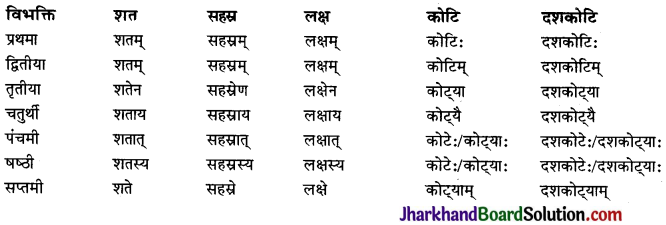संख्या जानने के लिए ‘कति’ (कितने) शब्द से प्रश्न बनाया जाता है। अतः विद्यार्थियों की सुविधा के लिए ‘कति’ शब्द के रूप दिये जा रहे हैं।

विभक्ति – कति (कितने)

प्रथमा – कति
द्वितीया – कति
तृतीया – कतिभिः
चतुर्थी – कतिभ्यः
पंचमी – कतिभ्यः
षष्ठी – कतीनाम्
सप्तमी – कतिषु

नोट – (i) ‘कति’ के रूप तीनों लिंगों में एक समान चलते हैं।
(ii) कति शब्द के रूप नित्य बहुवचनान्त बनते हैं।

अभ्यासः

प्रश्न 1.
अधोलिखित प्रश्नानां उत्तरस्य उचित विकल्प चित्वा लिखत –
(i) ‘2021’ इत्यस्य संस्कृतभाषायां रूप भवति
(क) एकविंशत्यधिकैकशतम्
(ख) एकविंशतिद्विशतम्
(ग) एकविंशतिशतम्
(घ) एकविंशत्यधिकद्विशतम्
उत्तरम् :
(ख) एकविंशतिद्विशतम्

(ii) लता समीपे ……………… (110) रूप्यकाणि सन्ति।
(क) दशीनशतम्
(ख) एकादशशतम्
(ग) दशाधिकशतानि
(घ) दशशतम्
उत्तरम् :
(ग) दशाधिकशतानि(iii) अस्माकं ग्रामे …………….. (3000) जनाः सन्ति ।
(क) त्रिसहस्र
(ख) त्रिसहस्राणि
(ग) त्रिसहस्रनं
(घ) त्रिसहस्रस्य
उत्तरम् :
(ख) त्रिसहस्राणि

(iv) विद्यालये …………….. (500) छात्राः सन्ति।
(क) पञ्चदशशतम्
(ख) पञ्चाशत्
(ग) पञ्चाधिकशतं
(घ) पञ्चशतम्
उत्तरम् :
(घ) पञ्चशतम्

(v) सीता तस्मै ……………… (175) रूप्यकाणि ददाति।
(क) पञ्चसप्तत्यधिकशतानि
(ख) पञ्चदशशतानि
(ग) पञ्चसप्ततिशतानि
(घ) पञ्चसप्तदशशतानि
उत्तरम् :
(क) पञ्चसप्तत्यधिकशतानि

प्रश्न 2.
निम्नलिखित अंकी संस्कृते लिखत –
(क) 103,
(ख) 180
उत्तरम् :
(क) व्यधिकशतम्,
(ख) अशीत्यधिकैकशतम्।

प्रश्न 3.
निम्नलिखितौ अंकी संस्कृते लिखत –
(क) 117
(ख) 180
उत्तरम् :
(क) सप्तदशाधिकैकशतम्,
(ख) अशीत्यधिकैकशतम्।प्रश्न 4.
निम्नलिखितौ अंकी संस्कृते लिखत –
(क) 401
(ख) 183
उत्तरम् :
(क) एकाधिकचतुः शतम्,
(ख) त्र्यशीत्यधिकैकशतम्।

प्रश्न 5.
निम्नलिखितौ अंकी संस्कृते लिखत –
(क) 115
(ख) 231
उत्तरम् :
(क) पञ्चदशाधिकशतम्,
(ख) एकत्रिंशदधिकद्विशतम्।

प्रश्न 6.
अधोलिखितसंख्याः संस्कृतभाषायां लिखत।
उत्तरम् :
188 – अष्टाशीत्यधिकशतम्।
159 – एकोनषट्यधिकशतम्।
300 – त्रिशतम्।
1965 – पञ्चषष्ट्यधिकैकोनविंशतिशतम्।
2000 – द्विसहस्रम्।
101 – एकाधिकशतम्।
140 – चत्वारिंशदधिकशतम्।
165 – पञ्चषष्ट्यधिकशतम्।
177 – सप्तसप्तत्यधिकशतम्।प्रश्न 7.
अधोलिखित संख्याशब्दान् अङ्केषु लिखत।
उत्तरम् :
एकपञ्चाशदधिकशतम् – 151
एकाधिकद्विशतम् – 201
एकत्रिंशदधिकत्रिशतम् – 331
पञ्चचत्वारिंशदधिकचतुः शतम् – 445
दशाधिकपञ्चशतम् – 510

प्रश्न 8.
अधोलिखितसंख्याशब्दान् अङ्केषु लिखत।
उत्तरम् :
पञ्चविंशत्यधिकत्रयोदशशतम्
1325 चतुःशतम् एकसप्तत्यधिकशतम्
171 नवाधिकशतम्
109 द्विशतम्
सप्तदशाधिकपञ्चशतम्
517 त्रिसप्तत्यधिकषट्शतम्

प्रश्न 9.
अधोलिखित संख्याशब्दान् संस्कृत भाषायां लिखत।
उत्तरम् :
620 – विंशत्यधिकषट्शतम्
751 – एकपञ्चाशदधिकसप्तशतम्
1021 – एकविंशत्यधिकसहस्रम्
1513 – त्रयोदशाधिकपञ्चदशशतम्
1966 – षट्पष्ट्यधिकैकोनविंशतिशतम्प्रश्न 10.
अधोलिखित संख्याशब्दान् संस्कृत भाषायां लिखत।
उत्तरम् :
2000 – द्विसहस्रम्
2020 – विंशत्यधिकद्विसहस्त्रम्
5200 – द्विशताधिकपञ्चसहस्त्रम्
10000 – दशसहस्रम्
1500000 – पञ्चदशलक्षम्

प्रश्न 11.
निम्नलिखितसंख्याः संस्कृतभाषायां लिखत।
उत्तरम् :
111 – एकादशाधिकशतम्
125 – पञ्चविंशत्यधिकशतम्
131 – एकत्रिंशदधिकशतम्
501 – एकाधिकपञ्चशतम्
650 – पञ्चाशदधिकष्टशतम्

प्रश्न 12.
अधोलिखित संख्या शब्दान् अङ्केषु लिखत।
उत्तरम् :
पञ्चसप्तत्यधिकसप्तशतम् – 775
चत्वरिंशदधिकाष्टशतम् – 840
त्रिंशदधिकनवशतम् – 930
पञ्चषष्ट्यधिकनवशतम् – 965
एकाधिकसहस्रम् – 1001
अष्टाधिकसहस्रम् – 1008प्रश्न 13.
अधोलिखित संख्या शब्दान् अङ्केषु लिखत।
उत्तरम् :
शताधिकसहस्रम् – 1100
एकादशाधिकैकादशशतम् – 1111
पंचविंशदधिकचतुर्दशशतम् – 1425
पंचाशित्यधिकपञ्चदशशतम् – 1585
चतुरशित्यधिकाष्टादशशतम् – 1884
एकोनद्विसहस्रम् – 1999

## JAC Class 10 Sanskrit व्याकरणम् धातुरूप-प्रकरणम्

Jharkhand Board JAC Class 10 Sanskrit Solutions व्याकरणम् धातुरूप-प्रकरणम् Questions and Answers, Notes Pdf.

## JAC Board Class 10th Sanskrit व्याकरणम् धातुरूप-प्रकरणम्

धातु – क्रिया के मूल रूप को धातु कहते हैं। जैसे – पठ् (पढ़ना), गम् (जाना), हस् (हँसना), क्रीड् (खेलना) आदि। धातु दो प्रकार की होती हैं –
1. सकर्मक – जिन धातुओं के साथ अपना कर्म रहता है वे सकर्मक धातुएँ होती हैं, जैसे- पठ्, गम आदि।
2. अकर्मक – जिन धातुओं के साथ अपना कर्म नहीं रहता, वे अकर्मक धातुएँ होती हैं, जैसे- वृद्धि, नर्तन, निद्रा आदि।

पद – संस्कृत भाषा में तीन पद होते हैं –

1. परस्मैपद – जिन क्रियाओं का फल कर्ता को प्राप्त न होकर किसी अन्य व्यक्ति को मिलता है वह परस्मैपद धातु कहलाती है।
2. आत्मनेपद – जिन क्रियाओं का फल कर्ता स्वयं प्राप्त करता है वे आत्मनेपदी धातु होती हैं।।।
3. उभयपद – जो धातुएँ परस्मैपद तथा आत्मनेपद दोनों में प्रयोग की जाती हैं उन्हें उभयपदी धातु कहते हैं। पाठ्यक्रम में निर्धारित धातु रूप इस प्रकार हैं –

परस्मैपद धातव –
(i) भू, पठ्, हस्, वच्, लिख्, अस्, हन्, पा, नृत्, आप्, शक्, कृ, ज्ञा, चिन्त् तथा इनकी समानार्थक धातुएँ।
(ii) आत्मनेपद- सेव, लभ्, रुच्, मुद्, याच्।
(ii) उभयपद – नी, हृ, भज, पच्।गण-क्रिया को संस्कृत में ‘धातु’ कहते हैं। संस्कृत की समस्त धातुओं को 10 गणों में बाँटा गया है। इन गणों के नाम इनकी प्रथम धातु के आधार पर रखे गये हैं। गण तथा उनकी कुछ मुख्य धातुएँ इस प्रकार हैं –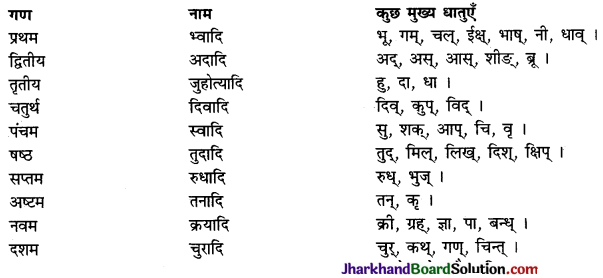लकार: – 1. लट् लकार-वर्तमान काल में लट् लकार का प्रयोग होता है। जिस काल में क्रिया का प्रारम्भ होना तथा चालू रहना सूचित होता हो और क्रिया की समाप्ति न पाई जाये, उसे वर्तमान काल कहते हैं ; जैसे – ‘रामः पुस्तकं पठति’-राम पुस्तक पढ़ता है, यहाँ पर पठन क्रिया प्रचलित है तथा समाप्ति का बोध नहीं होता। अतः वर्तमान काल है। इसी प्रकार ‘सः लिखति’ (वह लिखता है), ‘ते गच्छन्ति’ (वे जाते हैं) आदि वाक्यों में भी वर्तमान काल समझना चाहिए।

2. लृट् लकार – लृट् लकार का प्रयोग भविष्यत् काल में होता है अर्थात् क्रिया का वह काल जिसमें क्रिया का प्रारम्भ होना तो न पाया जाये किन्तु उसका आगे होना पाया जाये, उसे भविष्यत् काल कहते हैं; जैसे –

‘स: वाराणसीं गमिष्यति’ (वह वाराणसी जायेगा)। इस वाक्य में गमन क्रिया का आगे होना पाया जाता है। अतः यह भविष्यत् काल है। इसके लिए लट् लकार का प्रयोग किया जाता है।

3. लङ् लकार – अनद्यतन भूतकाल में लङ् लकार का प्रयोग होता है। अनद्यतन भूत वह काल है जो आज का न हो, अर्थात् आज बारह बजे रात से पूर्व का काल अथवा आज प्रातः से पूर्व का समय; जैसे . देवदत्तः विद्यालयम् अगच्छत्। (देवदत्त विद्यालय गया।)

4. लोट् लकार – लोट् लकार का प्रयोग आज्ञा देने के अर्थ में होता है। अतः सामान्य बोलचाल की भाषा में इसे ‘आज्ञा काल’ कहते हैं। यथा-‘देवदत्तः गृहं गच्छतु। (देवदत्त घर जाये)। यहाँ देवदत्त को घर जाने की आज्ञा दी गई है। अतः लोट् लकार का प्रयोग हुआ है।5. विधिलिङ् – इस लकार का प्रयोग विधि, निमन्त्रण, आमन्त्रण, अभीष्ट, सलाह और प्रार्थना आदि अर्थों में किया जाता है। सामान्यतः यह ‘चाहिए’ वाले वाक्य में प्रयुक्त होता है। जैसे – ‘देवदत्तः इदं कार्य कुर्यात्।’ (देवदत्त को यह कार्य करना चाहिए), ‘स: गृहं गच्छेत्’ (उसे घर जाना चाहिए)।

धातु-रूप

1. भू(होना) धातु (वर्तमान काल) परस्मैपदी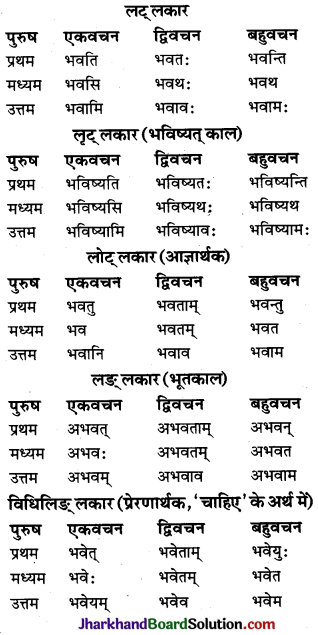2. गम् (गच्छ्) (जाना) धातु परस्मैपदी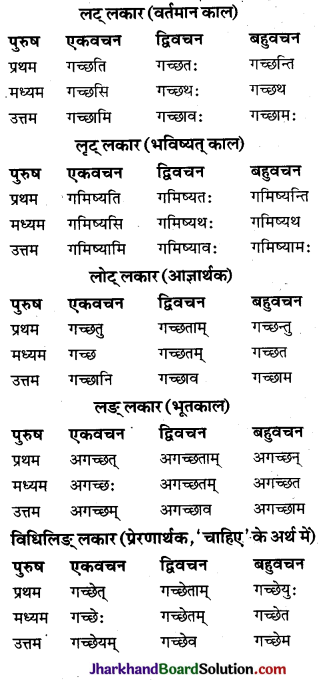3. इष (इच्छ) (इच्छा करना) धातु परस्मैपदी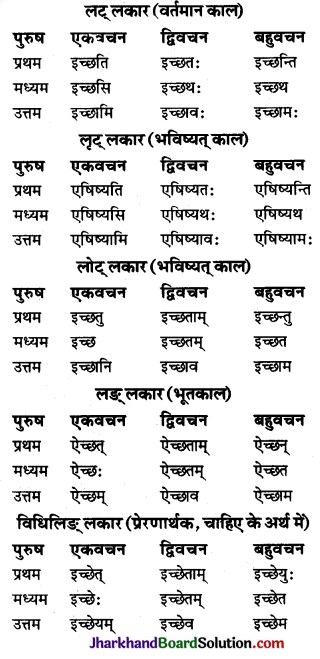4. प्रच्छ (पृच्छ) (पूछना) धातु परस्मैपदी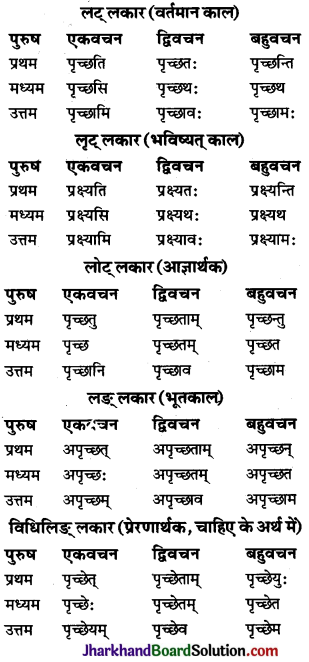5. लिख (लिखना) परस्मैपदी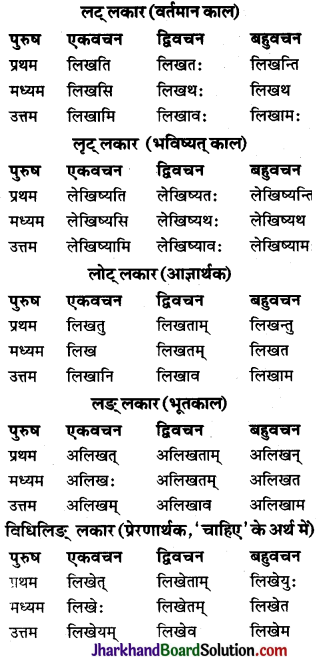6. हन् (मारना) धातु परस्मैपदी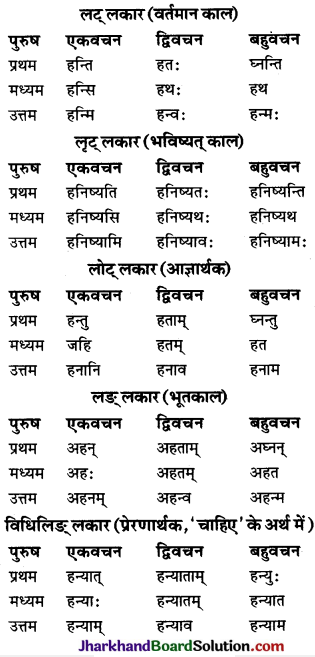7. भी (डरना) धातु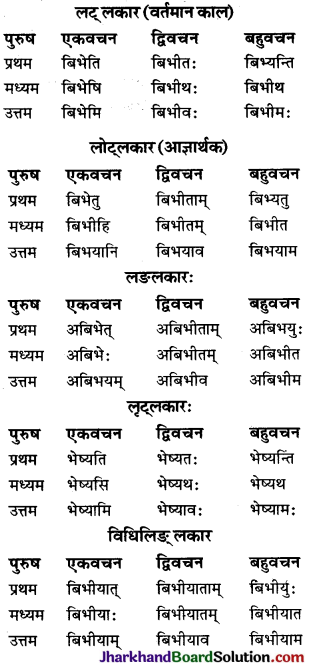8. दा (देना) धातु परस्मैपदी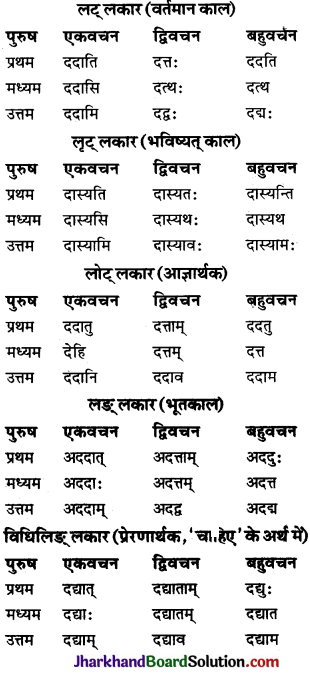9. नृत् (नाचना) धातु परस्मैपदी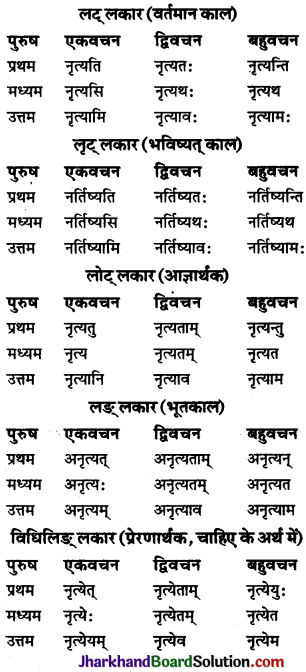10. जन् (पैदा होना) धातुः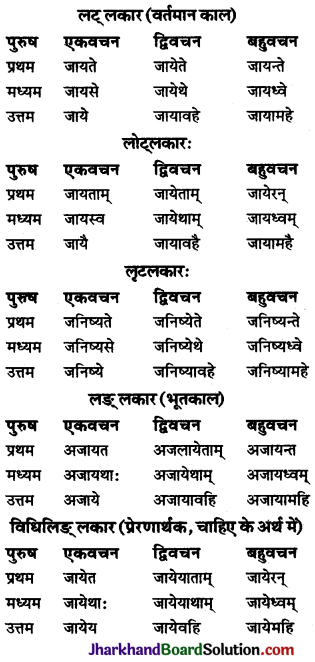11. कृ (कर) (करना) धातु परस्मैपदी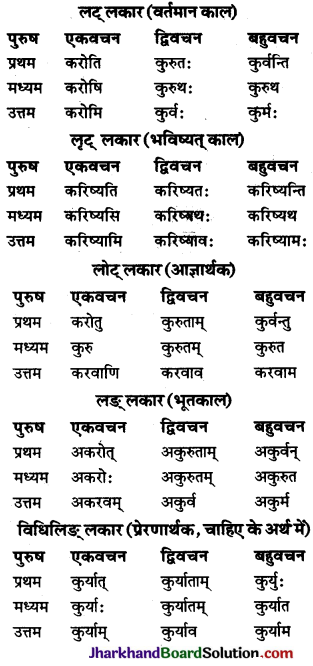12. की (द्रव्यविनिमये) धातु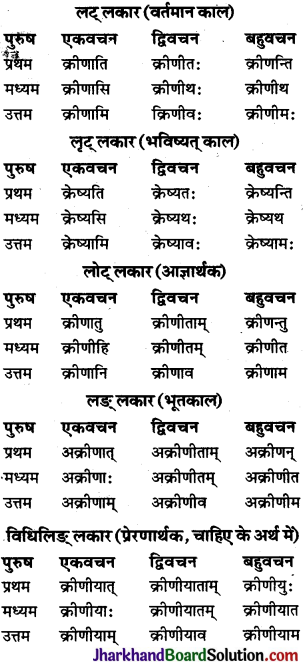13. क्रीड् (खेलना) परस्मैपदी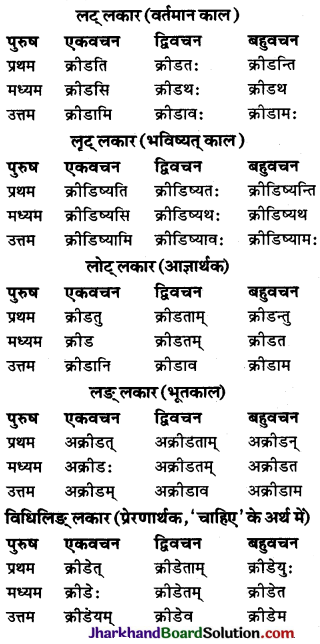14. चिन्त् (चिन्तय) (सोचना) धातु परस्मैपदी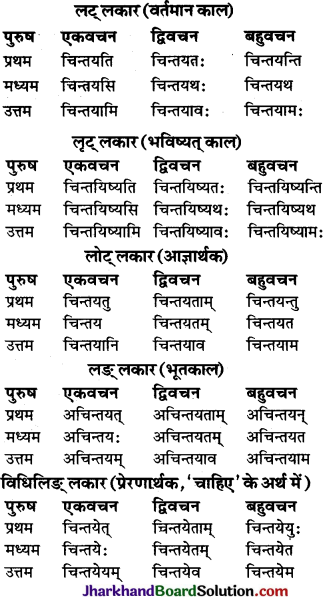15. त्यज् (हानौ) धातुः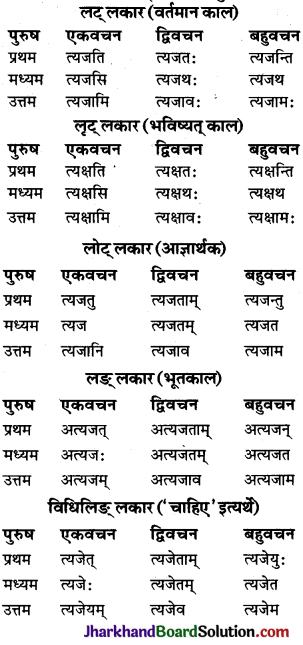16. सेव् (सेवा करना) धातु आत्मनेपदी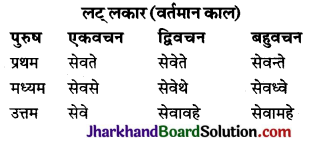17. लभ् (पाना) धातु आत्मनेपदी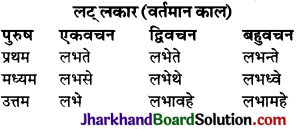अभ्यासः

प्रश्न 1.
अधोलिखित वाक्येषु सम्यक् विकल्प चिनुत् –
1. ‘भाष्’ धातोः लङ्लकारः उत्तम पुरुष, द्विवचने रूपं भवति –
(क) अभाषत
(ख) अभाषध्वम्
(ग) अभाषावहि
(घ) अभाषथाः
उत्तरम् :
(ग) अभाषावहि2. ‘वद्’ धातोः लङ्लकार उत्तमपुरुष एकवचने रूपं भवति –
(क) अवदम्
(ख) वदति
(ग) वदथः
(घ) वदिष्यथः।
उत्तरम् :
(क) अवदम्

3. इष् धातोः लट्लकारस्य उत्तमपुरुषस्यैकवचने रूपं भविष्यति –
(क) इच्छसि
(ख) इच्छामः
(ग) एषिष्यामि
(घ) इच्छामि।
उत्तरम् :
(घ) इच्छामि।

4. प्रच्छ धातोः लुट्लकारस्य प्रथमपुरुषस्य बहुवचने रूपं भविष्यति –
(क) प्रक्ष्यन्ति
(ख) प्रक्ष्यसि
(ग) प्रक्ष्यतः
(घ) प्रक्ष्यामः
उत्तरम् :
(क) प्रक्ष्यन्ति5. हन् धातोः लोट्लकारस्य मध्यमपुरुषस्यैकवचने रूपं भविष्यति –
(क) हनतु
(ख) जहि
(ग) हत
(घ) हतम्
उत्तरम् :
(ख) जहि

6. भी धातोः लङलकारस्य प्रथमपुरुषस्य बहुवचने रूपं भविष्यति –
(क), अबिभेत्
(ख) अबिभेः
(ग) अबिभीत
(घ) अबिभयुः
उत्तरम् :
(घ) अबिभयुः

7. दा धातोः विधिलिङ्लकारे मध्यमपुरुषैकवचने रूपं भविष्यति –
(क) दद्युः
(ख) दद्यात्
(ग) दद्याः
(घ) दद्याव
उत्तरम् :
(ग) दद्याः

8. नृत् धातोः लुट्लकारस्य उत्तमपुरुषस्य बहुवचने रूपं भविष्यति –
(क) नृत्यन्ति
(ख) नृत्यथ
(ग) नर्तिष्यामः
(घ) नृत्येमः
उत्तरम् :
(ग) नर्तिष्यामः9. जन् धातोः लङलकारस्य मध्यमपुरुषैकवचने रूपं भविष्यति –
(क) जाये
(ख) जायै
(ग) अजाये
(घ) जायेम
उत्तरम् :
(ग) अजाये

10. क्री धातोः लट्लकारस्य उत्तमपुरुषस्य बहुवचने रूपं भविष्यति –
(क) क्रीणीमः
(ख) क्रेष्यामः।
(ग) क्रीणाम
(घ) अक्रीणीम
उत्तरम् :
(क) क्रीणीमः

11. चिन्त् धातोः विधिलिङलकारस्य उत्तमपुरुषस्यैकवचने रूपं भविष्यति –
(क) चिन्तयेत्
(ख) चिन्तयेयम्
(ग) चिन्तयानि
(घ) चिन्तयेः
उत्तरम् :
(ख) चिन्तयेयम्12. त्यज़ धातोः लट्लकारस्य मध्यमपुरुषस्य बहवचने रूपं भविष्यति –
(क) त्यजथ
(ख) त्यजत
(ग) त्यक्ष्यथ
(घ) त्यजेत
उत्तरम् :
(ग) त्यक्ष्यथ

प्रश्न 2.
निर्देशानुसारं धातुरूपं लिखित्वा रिक्तस्थानानि पूरयत-(निर्देशानुसार धातुरूप लिखकर रिक्तस्थानों की पूर्ति कीजिए)
1. महान्तं विकार …………………. (कृ, लट् लकार)
2. अयं योगी परिव्राजकः ……………. (क्रीड्, लुट लकार)
3. त्वं मां खादितुम् ………………. (इच्छ, लृट् लकार)
4. जनाः मयि स्नानं ……………….. (कृ, लट् लकार)
उत्तराणि-
1. करिष्यति
2. क्रीडिष्यति
3. एषिष्यसि
4. करिष्यन्ति।

प्रश्न 3.
अधोलिखितेषु वाक्यानां रिक्तस्थानेषु निर्देशानुसारं धातु-रूपं लिखत –
(निम्नलिखित वाक्यों के रिक्तस्थानों में निर्देशानुसार धातुरूप लिखिए)
1. सिंहः एकां महतीं गुहां दृष्ट्वा …………… । (चिन्त, लङ्लकार)
2. सिंहः सहसा शृगालस्य आह्वानम् ……………… (कृ, लङ् लकार)
4. अन्येऽपि पशवः भयभीताः ……………… (भू, लङ्लकार)
5. चञ्चलः नदी जलम्………….. (पृच्छ, लङ्लकार)
6. नापिताः अस्यां रूढौ सहभागिताम्…………….. (त्यज, लङ् लकार)
उत्तराणि :
1. अचिन्तयत्
2. अकरोत्
3. अभवन्
4. अपृच्छत्
5. अत्यजन्।प्रश्न 4.
उचितधातुरूपैः वाक्यानि पूरयत –
(उचित धातु रूपों से वाक्यों को पूर्ण कीजिए-)
(i) विद्यार्थी ज्ञानं …………………………… (लभ-लट्लकारे, आत्मनेपदी)
(ii) श्वः मम मित्रं …………………………….. (गम्-लुटलकारे)
(iii) शिक्षकः विद्यालये किं …………. (कृ-लुट्लकारे)
(iv) शिष्यः गुरून् …………………….. (सेव्-लोट्लकारे)
(v) श्व: भौमवासरः ………. (भू लुट्लकारे)
(vi) सः संगीतमाध्यमेन निर्माणम् ………… (कृ-लङ्लकारे)
(vii) मन्द-मन्दम् पवनः मधुरं संगीतं ………….. (जन् लट्लकारे)
(viii) आयुष्मान् …………………… (भू-लोट्लकारे)
(ix) रीना शीघ्रम् उन्नति ……………….. (कृ-लट्लकारे)
(x) तौ गुरुम् ………………… (सेव्-लट्लकारे)
(xi) वयं विद्यालयं ………………. (गम्- लट्लकारे)
उत्तरम् :
(i) लभते
(ii) गमिष्यति
(iii) करोति
(iv) सेवताम्
(v) भविष्यति
(vi) अकरोत्
(vii) जनयति
(viii) भव
(ix) करोति
(x) सेवेते
(xi) गमिष्यामः

प्रश्न 5.
उचितधातुरूपैः वाक्यानि पूरयत – (उचित धातुरूपों से वाक्यों को पूर्ण कीजिए)
(i) रमा सीता च श्वः तत्र …………… (गम्)
(ii) सः प्रातः व्यायामं ……… (कृ)
(iii) ह्यः अहं विद्यालयम् …………….. (गम्)
(iv) सीता ह्यः मातुः पत्रम् …………… (लभ)
उत्तरम् :
(i) गमिष्यतः
(ii) करोति
(iii) अगच्छम्
(iv) अलभत।प्रश्न 6.
निर्देशानुसारं धातु-रूपं लिखत –
(निर्देशानुसार धातुरूप लिखिए-)
1. भू (लोट् लकार मध्यम पुरुष बहुवचन)
2. दृश् (लट् लकार मध्यम पुरुष एकवचन)
3. गम् (विधिलिङ् लकार उत्तम पुरुष द्विवचन)
4. चिन्त् (लट् लकार मध्यम पुरुष द्विवचन)
5. गम् (लट् लकार मध्यम पुरुष द्विवचन)
6. सेव् (लट् लकार मध्यम पुरुष बहुवचन)
7. कृ (विधिलिङ् लकार उत्तम पुरुष एकवचन)
8. जन् (लङ् लकार प्रथम पुरुष बहुवचन)
9. क्रीड् (लोट् लकार मध्यम पुरुष द्विवचन)
10. लिख (लट् लकार उत्तम पुरुष एकवचन)
11. इष् (इच्छ्) (लुट् लकार मध्यम पुरुष बहुवचन)
उत्तराणि :
1. भवत
2. पश्यसि
3. गच्छेव
4. चिन्तयथः
5. गमिष्यथ:
6. सेवध्वे
7. कुर्याम्
8. अजायन्त
9. क्रीडतम्
10. लेखिष्यामि
11. एषिष्यथ।

प्रश्न 7.
अधोलिखित पदेषु धातु-लकार-पुरुष वचनानां निर्देशं कुरुत –
(i) क्रीडति
(ii) करोमि
(iii) जनिष्ये
उत्तरम् :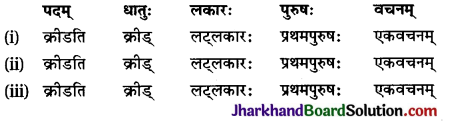प्रश्न 8.
अधोलिखित पदेषु धातु-लकार-पुरुष वचनानां निर्देशं कुरुत –
(i) भवथ
(ii) गच्छामः
(iii) इच्छसि
उत्तरम् :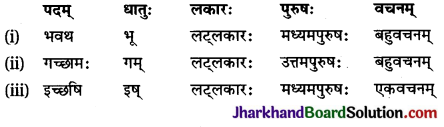प्रश्न 9.
अधोलिखित पदेषु धातु-लकार-पुरुष वचनानां निर्देशं कुरुत –
(i) पृच्छन्ति
(ii) अपृच्छत्
(iii) लिखन्ति
उत्तरम् :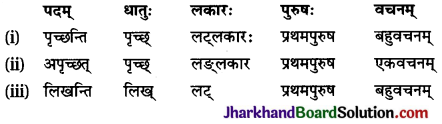प्रश्न 10.
अधोलिखित पदेषु धातु-लकार-पुरुष वचनानां निर्देशं कुरुत –
(i) लिखेम
(ii) हनिष्यामः
(iii) हन्याः
उत्तरम् :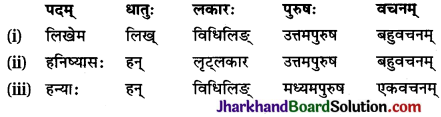प्रश्न 11.
अधोलिखित पदेषु धातु-लकार-पुरुष वचनानां निर्देशं कुरुत
(i) बिभ्यन्ति
(ii) भेस्यथ
उत्तरम् :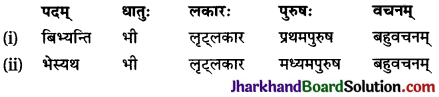प्रश्न 12.
अधोलिखित पदेषु धातु-लकार-पुरुष वचनानां निर्देशं कुरुत –
(i) ददति
(ii) अदत्ताम्
(iii) नर्तिस्यथः
उत्तरम् :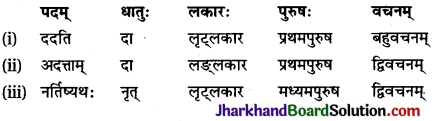प्रश्न 13.
अधोलिखित पदेषु धातु-लकार-पुरुष वचनानां निर्देशं कुरुत –
(i) जायसे
(ii) कुरुथः
(iii) कुर्याम्
उत्तरम् :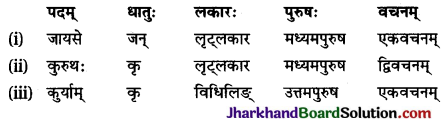प्रश्न 14.
अधोलिखित पदेषु धातु-लकार-पुरुष वचनानां निर्देशं कुरुत –
(i) क्रेष्यथ:
(ii) क्रीडानि
(iii) क्रीडेयम्
उत्तरम् :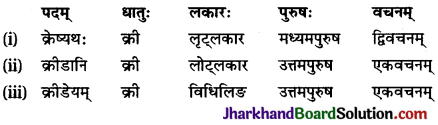प्रश्न 15.
अधोलिखित पदेषु धातु-लकार-पुरुष वचनानां निर्देशं कुरुत –
(i) चिन्तयिष्यथ:
(ii) त्यजताम्
(iii) सेवते
उत्तरम् :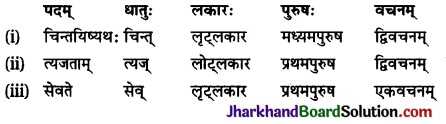## JAC Class 9 Maths Solutions Chapter 1 Number Systems Ex 1.4

Jharkhand Board JAC Class 9 Maths Solutions Chapter 1 Number Systems Ex 1.4 Textbook Exercise Questions and Answers.

## JAC Board Class 9th Maths Solutions Chapter 1 Number Systems Exercise 1.4

Question 1.
Visualise 3.765 on the number line using successive magnification.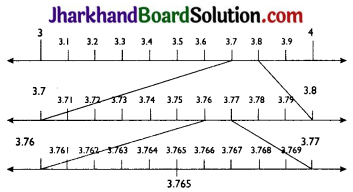Question 2.
Visualize 4.26 on the number line, up to 4 decimal places.
$$4 . \overline{26}$$ = 4.2626…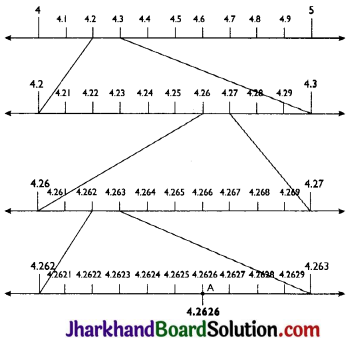## JAC Class 9 Maths Solutions Chapter 1 Number Systems Ex 1.3

Jharkhand Board JAC Class 9 Maths Solutions Chapter 1 Number Systems Ex 1.3 Textbook Exercise Questions and Answers.

## JAC Board Class 9th Maths Solutions Chapter 1 Number Systems Exercise 1.3

Question 1.
Write the following in decimal form and say what kind of decimal expansion each has:
(i) $$\frac{36}{100}$$
(ii) $$\frac{1}{11}$$
(iii) $$4 \frac{1}{8}$$
(iv) $$\frac{3}{13}$$
(v) $$\frac{2}{11}$$
(vi) $$\frac{329}{400}$$
(i) $$\frac{36}{100}$$ = 0.36 (Terminating)

(ii) $$\frac{1}{11}$$ = 0.09090909… = $$0 . \overline{09}$$ (Non-terminating and repeating)

(iii) $$4 \frac{1}{8}$$ = $$\frac{33}{8}$$ =4.125 (Terminating)

(iv) $$\frac{3}{13}$$ = 0.230769230769… = $$0 . \overline{230769}$$ (Non-terminating and repeating)

(v) $$\frac{2}{11}$$ = 0.181818181818… = $$0 . \overline{18}$$ (Non-terminating and repeating)

(vi) $$\frac{329}{400}$$ = 0.8225 (Terminating)

Question 2.
You know that $$\frac{1}{7}$$ = 0.142857. Can you predict what the decimal expansion of $$\frac{2}{7}, \frac{3}{7}, \frac{4}{7}, \frac{5}{7}, \frac{6}{7}, \frac{3}{7}, \frac{4}{7}, \frac{5}{7}, \frac{6}{7}$$ are without actually doing the long division? If so, how?
[Hint: Study the remainders while finding the value of $$\frac{1}{7}$$ carefully.]
Yes, We can do this by:

$$\frac{2}{7}=2 \times \frac{1}{7}=2 \times 0 . \overline{142857}=0 . \overline{285714}$$
$$\frac{3}{7}=3 \times \frac{1}{7}=3 \times 0 . \overline{142857}=0 . \overline{428571}$$
$$\frac{4}{7}=4 \times \frac{1}{7}=4 \times 0 . \overline{142857}=0 . \overline{571428}$$
$$\frac{5}{7}=5 \times \frac{1}{7}=5 \times 0 . \overline{142857}=0 . \overline{714285}$$
$$\frac{6}{7}=6 \times \frac{1}{7}=6 \times 0 . \overline{142857}=0 . \overline{857142}$$Question 3.
Express the following in the form p/q, where p and q are integers and q ≠ 0.
(i) $$0 . \overline{6}$$
(ii) $$0 . 4 \overline{7}$$
(iii) $$0 . \overline{001}$$
(i) $$0 . \overline{6}$$ = 0.666…
Let x = 0.666…
∴ 10x = 6.66…
∴ 10x = 6 + 0.66…
∴ 10x = 6 + 0.666…
∴ 10x = 6 + x
∴ 9x = 6
x = $$\frac{2}{3}$$

(ii) $$0 . 4 \overline{7}$$ = 0.4777… = $$\frac{4}{10}$$ + $$\frac{0.777}{10}$$
Let x = 0.777…
∴ 10x = 7.77…
∴ 10x = 7.777…
∴ 10x = 7 + 0.777…
∴ 10x = 7 + x
∴ x = $$\frac{7}{9}$$
$$0 . 4 \overline{7}$$ = $$\frac{4}{10}$$ + $$\frac{0.777}{10}$$ = $$\frac{4}{10}$$ + $$\frac{7}{90}$$
= $$\frac{36}{90}$$ + $$\frac{7}{90}$$ = $$\frac{43}{90}$$

(iii) $$0 . \overline{001}$$ =0.001001…
Let x = 0.001001…
∴ 1000x = 1.001….
∴ 1000x = 1.001001…
∴ 1000x = 1 + 0.001001 …
∴ l000x= 1 + X
∴ 999x = 1
x = $$\frac{1}{999}$$

Question 4.
Express 0.99999…in the form $$\frac{p}{q}$$. Are you surprised by your answer? With your teacher and classmates discuss why the answer makes sense.
Let x = 0.9999…
10x = 9.999…
∴ 10x = 9.9999…
∴ 10x = 9 + 0.9999…
∴ 10x = 9 + x
∴ 9x = 9
x = 1
The difference between 1 and 0.999999 is 0.000001 which is negligible. Thus, 0.999… is too much near 1. Therefore, the answer 1 can be justified.

Question 5.
What can the maximum number of digits be in the repeating block of digits in the decimal expansion of 1/17? Perform the division to check your answer.
$$\frac{1}{17}$$ = 0.05882352941176470588…
= $$0 . \overline{0588235294117647}$$
There are 16 digits in the repeating block of the decimal expansion of $$\frac{1}{17}$$

Question 6.
Look at several examples of rational numbers in the form $$\frac{p}{q}$$ (q ≠ 0), where p and q are integers with no common factors other than 1 and having terminating decimal representations (expansions). Can you guess what property q must satisfy?
We observe that when q is 2, 4, 5, 8, 10,… then the decimal expansion is terminating. For example:
$$\frac{1}{2}$$ = 0.5, denominator q = 21
$$\frac{4}{5}$$ = 0.8, denominator q = 51
We can observe that terminating decimal may be obtained in the situation where prime factorisation of the denominator of the given fractions has the power of 2 only or 5 only or both.Question 7.
Write three numbers whose decimal expansions are non-terminating non-recurring.
Three numbers whose decimal expansions are non-terminating non-recurring are:
(i) 0.303003000300003…
(ii) 0.505005000500005…
(iii) 0.7207200720007200007200000…

Question 8.
Find three different irrational numbers between the rational numbers $$\frac{5}{7}$$ and $$\frac{9}{11}$$.
$$\frac{5}{7}$$ = $$0 . \overline{714285}$$

$$\frac{9}{11}$$ = $$0 . \overline{81}$$
Three different irrational numbers are:
0.73073007300073000073…
0.75075007500075000075…
0.76076007600076000076…

Question 9.
Classify the following numbers as rational or irrational:
(i) $$\sqrt{23}$$
(ii) $$\sqrt{225}$$
(iii) 0.3796
(iv) 7.478478…
(v) 1.101001000100001…
(i) $$\sqrt{23}$$ = 4.79583152331…
Since the decimal expansion is non-terminating and non-recurring therefore, it is an irrational number.

(ii) $$\sqrt{225}$$ = 15 = $$\frac{15}{1}$$
The number is rational number as it can represented in $$\frac{p}{q}$$ form where p, q ∈ Z and q ≠ 0.

(iii) 0.3796
Since the decimal expansion is terminating therefore, it is a rational number.

(iv) 7.478478… = $$7 . \overline{478}$$
Since, this decimal expansion is non-terminating recurring, therefore, it is a rational number.

(v) 1.101001000100001…
Since the decimal expansion is non-terminating and non-repeating, therefore, it is an irrational number.

## JAC Class 9 Maths Solutions Chapter 1 Number Systems Ex 1.2

Jharkhand Board JAC Class 9 Maths Solutions Chapter 1 Number Systems Ex 1.2 Textbook Exercise Questions and Answers.

## JAC Board Class 9th Maths Solutions Chapter 1 Number Systems Exercise 1.2

Question 1.
State whether the following statements are true or false. Justify your Answers.
(i) Every irrational number is a real number.
(ii) Every point on the number line is of the form $$\sqrt{m}$$ , where m is a natural number.
(iii) Every real number is an irrational number.
(i) True, since the collection of real numbers is made up of rational and irrational numbers.
(ii) False, since negative numbers cannot be expressed as square roots.
(iii) False, as real numbers include both rational and irrational numbers. Therefore, every real number cannot be an irrational number, e.g. 2 is a real number but not an irrational number.

Question 2.
Are the square roots of all positive inte-gers irrational? If not, give an example of the square root of a number that is a rational number.
No, the square roots of all positive integers are not irrational. For example $$\sqrt{4}$$ = 2, which is rational number.Question 3.
Show how $$\sqrt{5}$$ can be represented on the number line.
Step 1: Let AB be a line segment of length 2 units on number line.
Step 2: At B, draw a perpendicular line BC of length 1 unit. Join CA.
Step 3: Now, ABC is a right angled triangle. Applying Pythagoras theorem,
AB2 + BC2 = CA2
⇒ 22 + 12 = CA2
⇒ CA2 = 5
⇒ CA = $$\sqrt{5}$$
Thus, CA is a line of length $$\sqrt{5}$$ units.

Step 4: Taking CA as a radius and A as a centre draw an arc touching the number line. The point D at which number line gets intersected by arc of length AC is at $$\sqrt{5}$$ distance from 0.
Thus, $$\sqrt{5}$$ is represented on the number line as shown in the figure.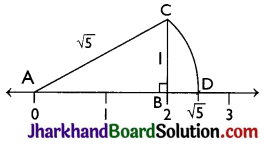## JAC Class 9 Maths Solutions Chapter 1 Number Systems Ex 1.1

Jharkhand Board JAC Class 9 Maths Solutions Chapter 1 Number Systems Ex 1.1 Textbook Exercise Questions and Answers.

## JAC Board Class 9th Maths Solutions Chapter 1 Number Systems Exercise 1.1

Question 1.
Is zero a rational number? Can you write it in the form $$\frac{p}{q}$$, where p and q are integers and q ≠ 0?
Yes, Zero is a rational number as it can be represented as $$\frac{0}{1}$$ or $$\frac{0}{2}$$ and so on.

Question 2.
Find six rational numbers between 3 and 4.
There are infinitely many rational numbers between 3 and 4.
3 and 4 can be represented as $$\frac{24}{8}$$ and $$\frac{32}{8}$$ respectively.
Therefore, six rational numbers between 3 and 4 are: $$\frac{25}{8}$$, $$\frac{26}{8}$$, $$\frac{27}{8}$$, $$\frac{28}{8}$$, $$\frac{29}{8}$$, $$\frac{30}{8}$$.Question 3.
Find five rational numbers between $$\frac{3}{5}$$ and $$\frac{4}{5}$$.
There are infinitely many rational numbers between $$\frac{3}{5}$$ and $$\frac{4}{5}$$
$$\frac{3}{5}=\frac{3 \times 6}{5 \times 6}=\frac{18}{30}$$
$$\frac{4}{5}=\frac{4 \times 6}{5 \times 6}=\frac{24}{30}$$
Therefore, five rational numbers between $$\frac{3}{5}$$ and $$\frac{4}{5}$$ are $$\frac{19}{30}$$, $$\frac{20}{30}$$, $$\frac{21}{30}$$, $$\frac{22}{30}$$, $$\frac{23}{30}$$.

Question 4.
State whether the following statements are true or false. Give reasons for your answers.
(i) Every natural number is a whole number.
(ii) Every integer is a whole number.
(iii) Every rational number is a whole number.
(i) True, since the collection of whole numbers contains all natural numbers.
(ii) False, as integers may be negative but whole numbers are always positive, e.g. – 1 is an integer but not a whole number.
(iii) False, as rational numbers may be fractional but whole numbers are not fractional, e.g. $$\frac{2}{3}$$ is a rational number but not a whole number.

## JAC Jharkhand Board Class 9th Maths Solutions in Hindi & English Medium

### JAC Board Class 9th Maths Solutions in English Medium

JAC Class 9 Maths Chapter 1 Number Systems

JAC Class 9 Maths Chapter 2 Polynomials

JAC Class 9 Maths Chapter 3 Coordinate Geometry

JAC Class 9 Maths Chapter 4 Linear Equations in Two Variables

JAC Class 9 Maths Chapter 5 Introduction to Euclid’s Geometry

JAC Class 9 Maths Chapter 6 Lines and Angles

JAC Class 9 Maths Chapter 7 Triangles

JAC Class 9 Maths Chapter 8 Quadrilaterals

JAC Class 9 Maths Chapter 9 Areas of Parallelograms and Triangles

JAC Class 9 Maths Chapter 10 Circles

JAC Class 9 Maths Chapter 11 Constructions

JAC Class 9 Maths Chapter 12 Heron’s Formula

JAC Class 9 Maths Chapter 13 Surface Areas and Volumes

JAC Class 9 Maths Chapter 14 Statistics

JAC Class 9 Maths Chapter 15 Probability

### JAC Board Class 9th Maths Solutions in Hindi Medium

JAC Class 9 Maths Chapter 1 संख्या पद्धति

JAC Class 9 Maths Chapter 2 बहुपद

JAC Class 9 Maths Chapter 3 निर्देशांक ज्यामिति

JAC Class 9 Maths Chapter 4 दो चरों वाले रैखिक समीकरण

JAC Class 9 Maths Chapter 5 युक्लिड के ज्यामिति का परिचय

JAC Class 9 Maths Chapter 6 रेखाएँ और कोण

JAC Class 9 Maths Chapter 7 त्रिभुज

JAC Class 9 Maths Chapter 8 चतुर्भुज

JAC Class 9 Maths Chapter 9 समान्तर चतुर्भुज और त्रिभुजों के क्षेत्रफल

JAC Class 9 Maths Chapter 10 वृत्त

JAC Class 9 Maths Chapter 11 रचनाएँ

JAC Class 9 Maths Chapter 12 हीरोन का सूत्र

JAC Class 9 Maths Chapter 13 पृष्ठीय क्षेत्रफल एवं आयतन

JAC Class 9 Maths Chapter 14 सांख्यिकी

JAC Class 9 Maths Chapter 15 प्रायिकता